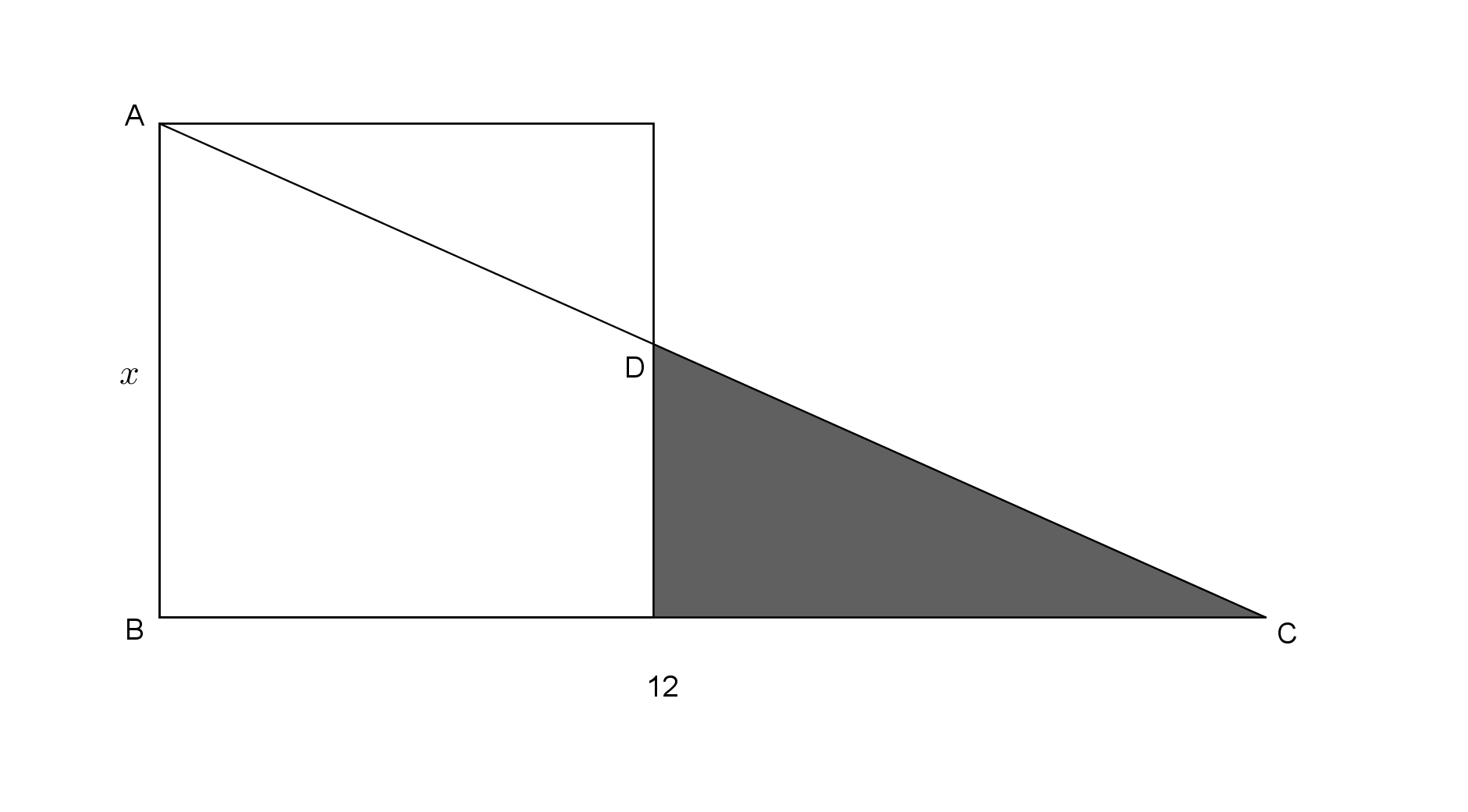# Its maximum area

Calculus Level 1A right triangle $ABC$ with side $AB = x$ and $BC = 12$ intersects a square with side $AB$ at the point $D$. Assuming $x$ is variable, find the value of $x\le 12$ that maximizes the shaded portion of the triangle.

×

Problem Loading...

Note Loading...

Set Loading...